# Identifying Denominations

Identifying Denominations
Go back to  'Money'

Emily's mom wanted to buy some groceries for the week. She drove her car to the supermarket and bought the items.

The shopkeeper gave her a shopping bill for $185. She handed her two$100 bills. The shopkeeper then handed over three $5 bills back to her. Emily wanted to know why some money was given back to her mom. She asked, "Mom, it is we who bought the groceries, so why is the shopkeeper paying us?" Her mom replied that the items they bought were for$185 and that the money that was returned was the amount remaining after the purchase.In this lesson, you will learn what is money, its monetary value, denominations meaning, adding and subtracting money, US currency notes and coins.

## Lesson Plan

 1 What is Identifying Denominations? 2 Important Notes on Identifying Denominations 3 Solved Examples on Identifying Denominations 4 Challenging Questions on Identifying Denominations 5 Interactive Questions on Identifying Denominations

## What Is Identifying Denominations?

Money is a common term used for currency.

It is exchanged between various people, used in trade, and deposited in banks.

Money, be it a printed currency note or a coin, as such does not have any value.

Its value is decided by the government or economists of a country who give it a particular value.

We buy goods or avail a service based on the numerical value denoted by money.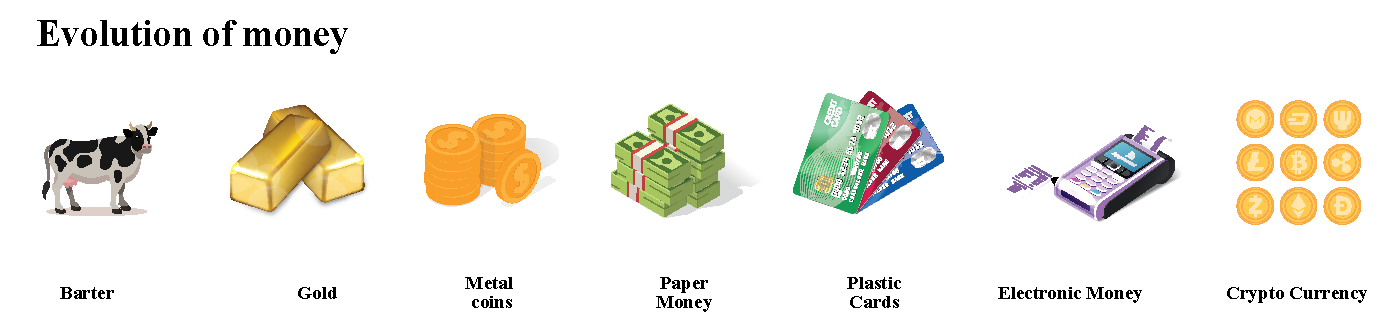Now let us learn about the history of money and what existed before money.

The usage of money has changed and evolved over the years.

The earliest system to buy and exchange goods was known as "Bartering," which involved the direct trade of goods and services.

Following the usage of bartering, the Chinese introduced a circular-shaped bronze object known as a "Coin." However, "Lydia" was the first region to manufacture coins.

The next transition by the Chinese was around 700 B.C when they introduced paper money. This is the modern-day currency that is in existence now.

Mode of Payment of Money

Currency notes are printed on paper and are mostly issued by the governments. These are still in use today.

In the earlier days, they were issued by banks and some private institutions.

Apart from that, we also have plastic cards (Credit and debit cards), electronic money transfer and crypto currency. A classic example for crypto currency is the "Bitcoin."

## What Do You Mean By Denomination of Money?

Splitting up a given number into various smaller numbers is called denomination.

Denomination meaning in the context of  money can be well understood by thinking of place values.

The place values are ones, tens, hundreds, thousands, and so on.

$1,$10, $100 are similar to place values of units, tens, and hundreds. There can be any number of one dollar, ten dollars, hundred dollars in a given amount. For example, we can say a hundred dollars comprises hundred$1 or ten $10 or one$100.

 \begin{align} 100 \:= \:(1 \times 100) \:or\: (10 \times 10)\: or \:(100 \times 1)\:\end{align}

These are the currency notes used in the United States.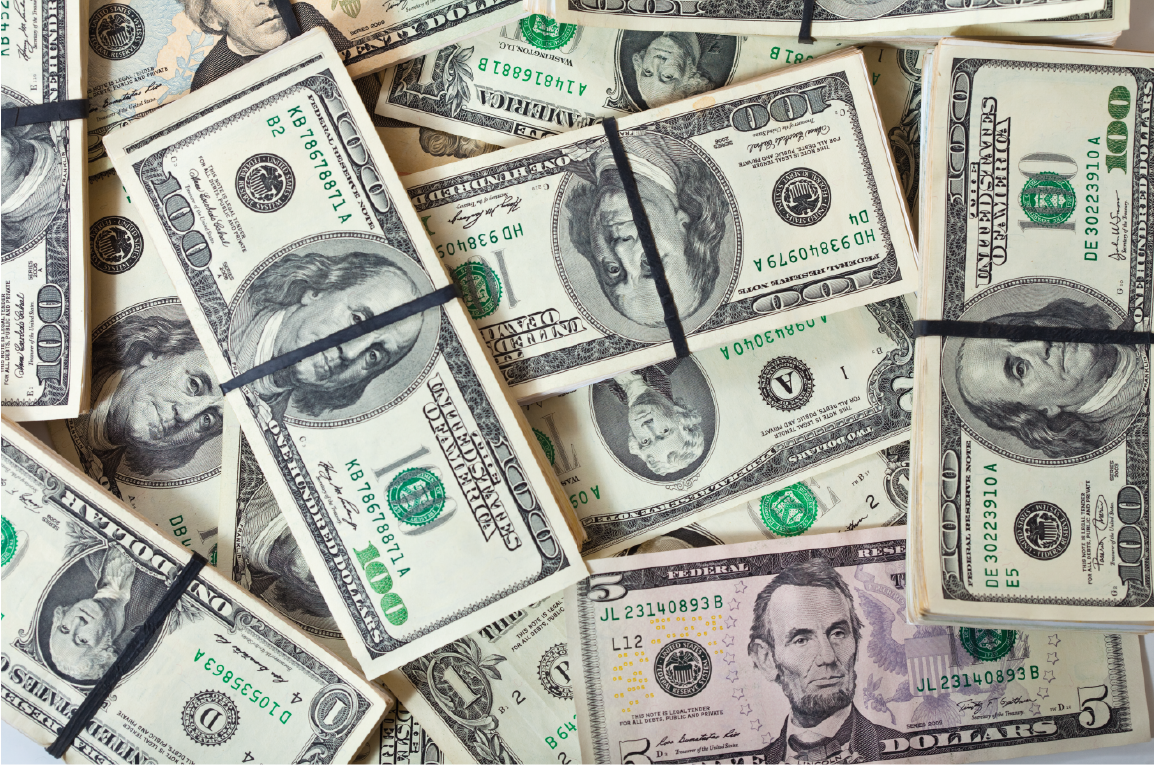The paper notes come in the following denominations:

• One dollar ($1) • Two dollars ($2)
• Five dollars ($5) • Ten dollars ($10)
• Twenty dollars ($20) • Fifty dollars ($50)
• Hundred dollars ($100) Apart from currency notes, we have circular-shaped objects called coins. Each coin denotes a specified number of cents. The coin denominations available are: • 1 cent (Penny) • 5 cents (Nickel), • 10 cents (One dime) • 25 cents (Quarter dollar) • 50 cents (Half dollar) • Dollar coins A cent looks like this.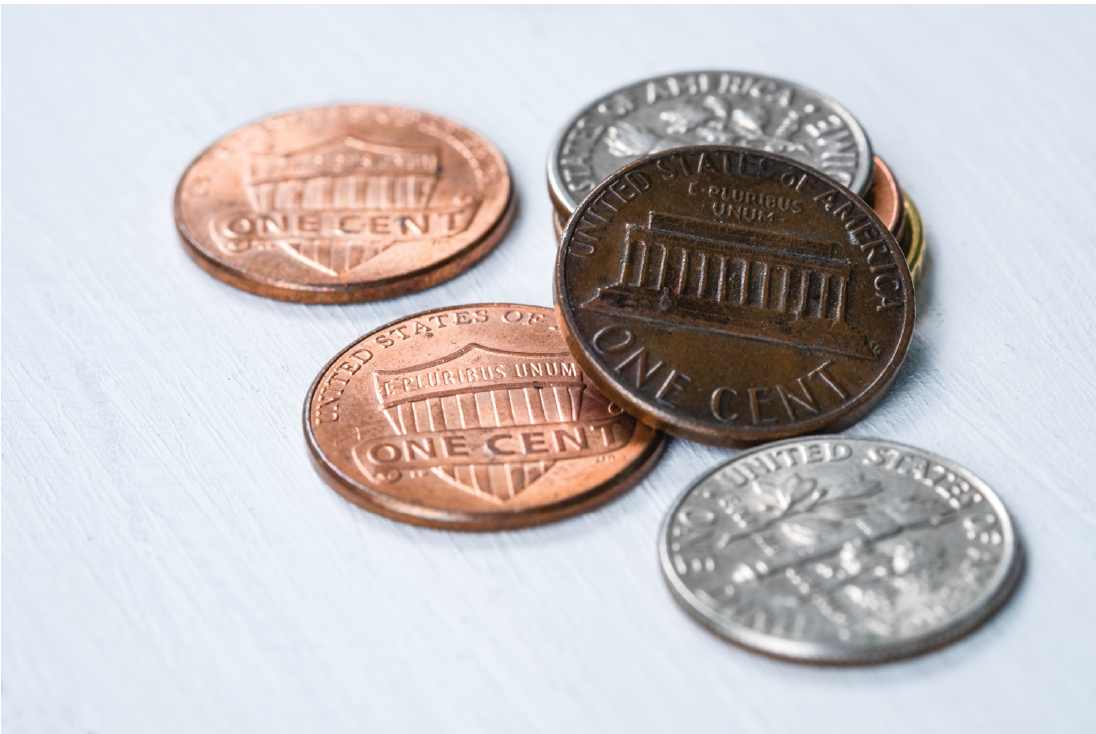100 cents make one dollar.  100 cents = 1 dollar Every bill and coin has a monetary value.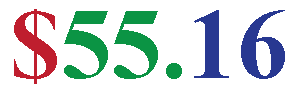This is read as 55 dollars and 16 cents. The symbol for US dollars and cents are shown below.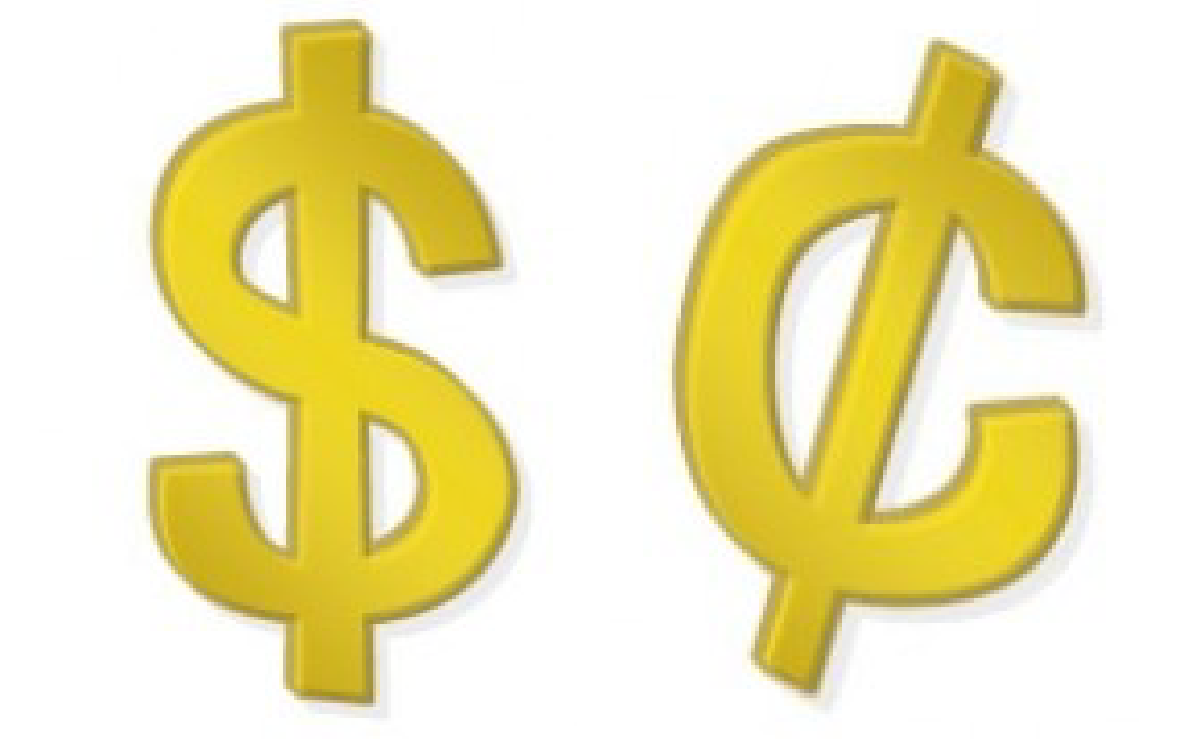## Addition and Subtraction With Denominations In this section we will glance through some arithmetic operations on money. When you buy goods, you will have to pay some amount to the party from whom you bought the goods. It is not possible to give the exact amount every time. Let us say you go to a shopping mall and buy things worth$465.

You have five $100 bills which add up to$500.

You have to pay $465, so what happens to the remaining amount? The shop keeper subtracts the amount to be paid by you from the total amount you've given. The extra amount is returned to you.$35 will be returned to you probably as three $10 and one$5.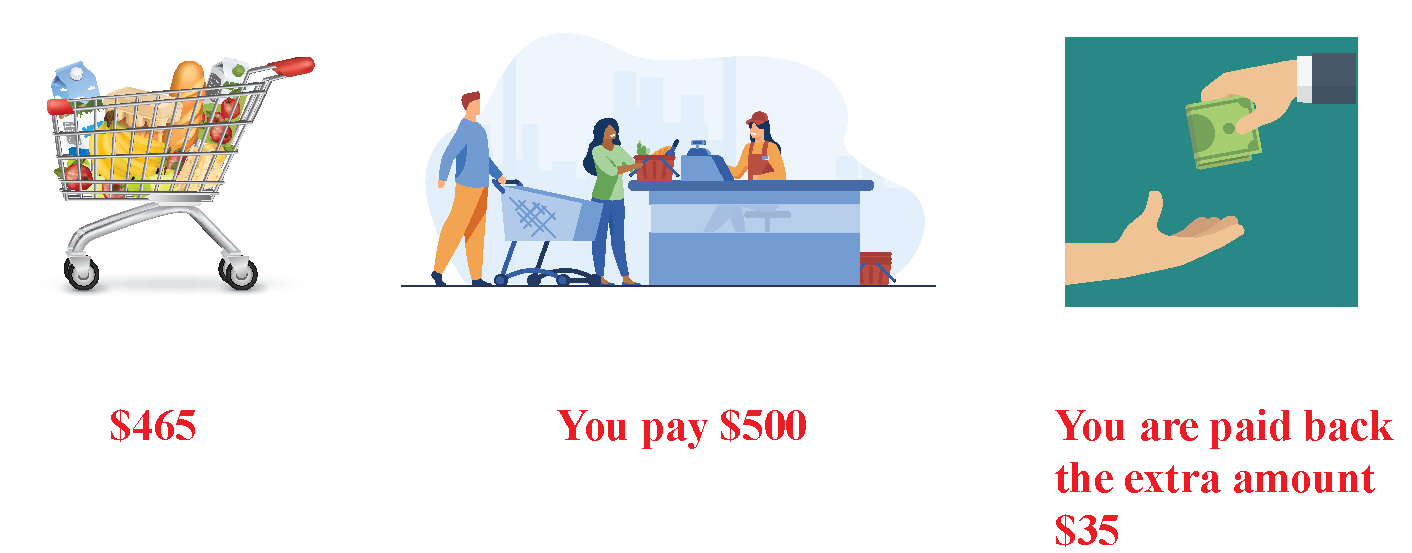In this case, you pay $500 and you are given back$500 - $465, which is equal to$35

In some instances, you may need to add the denominations of money.

If you have to buy some books worth $10 and a pack of pens worth$5, you can add both the denominations of money and pay \$15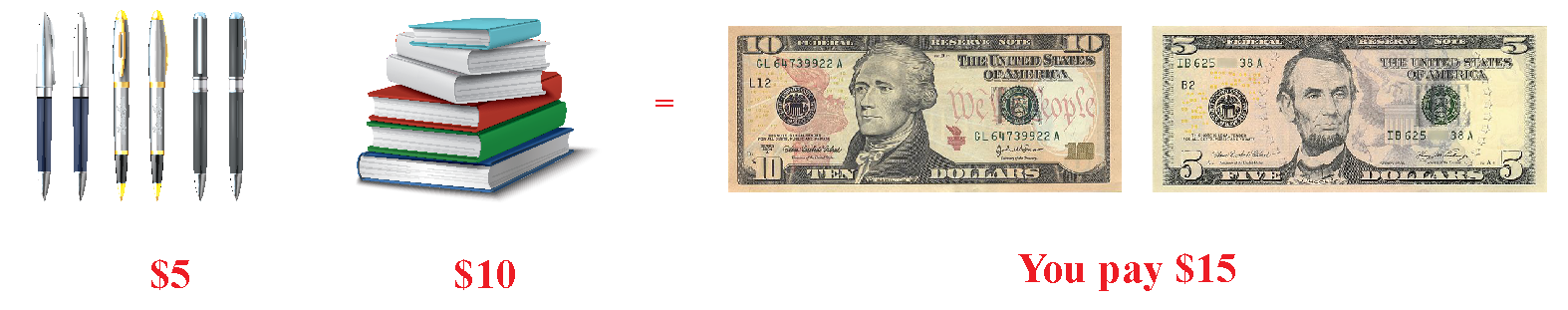The table below shows the amount of money and their denominations.

Amount of Money Denominations
\begin{align}2000\end{align} \begin{align}20 \times 100\end{align} or \begin{align}200 \times 10\end{align} or \begin{align}2000 \times 1\end{align}
\begin{align}500\end{align} \begin{align}5 \times 100\end{align} or \begin{align}50 \times 10\end{align} or \begin{align}500 \times 1\end{align}
\begin{align}100\end{align} \begin{align}1 \times 100\end{align} or \begin{align}10 \times 10\end{align} or \begin{align}20 \times 5\end{align} or \begin{align}100 \times 1\end{align}
\begin{align}10\end{align} \begin{align}1 \times 10\end{align} or \begin{align}10 \times 1\end{align} or \begin{align}2 \times 5\end{align}
Money
Kg | Worksheet 1
Money
Kg | Worksheet 2

More Important Topics
Numbers
Algebra
Geometry
Measurement
Money
Data
Trigonometry
Calculus